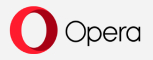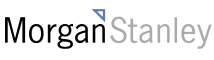New update is available. Click here to update.

# Mindbending Product

Contributed by
Dhruv Sharma
Easy0/40
20 mins
80 %19 upvotes+2 more

## Problem Statement

#### For Example:

``````For an array {1, 2, 3, 4, 5}:
The required product array generated from the given array is {120, 60, 40, 30, 24 }

This can be generated in the following manner:

For generating 120 we have 2 * 3 * 4 * 5 i.e. the product of other array elements except 1.
For generating 60 we have 1 * 3 * 4 * 5  i.e. the product of other array elements except 2.
For generating 40 we have 1 * 2 * 4 * 5  i.e. the product of other array elements except 3.
For generating 30 we have 1 * 2 * 3 * 5  i.e. the product of other array elements except 4.
For generating 24 we have 1 * 2 * 3 * 4  i.e. the product of other array elements except 5.
``````
Detailed explanation ( Input/output format, Notes, Images )
##### Constraints:
``````1 <= T <= 50
1 <= N <= 10
1 <= ARR [i] <= 20

Time Limit: 1 sec
``````
##### Sample Input 1:
``````2
5
10 3 5 6 2
2
12 20
``````
##### Sample Output 1:
``````180 600 360 300 900
20 12
``````
##### Explanation:
``````Test Case 1:
For the product array P,
At i=0 we have 3*5*6*2 = 180.
At i=1 we have 10*5*6*2 = 600.
At i=2 we have 10*3*6*2 = 360.
At i=3 we have 10*3*5*2 = 300.
At i=4 we have 10*3*5*6 = 900
So, the P array is 180 600 360 300 900

Test Case 2:
For the product array P,
At i=0, we have 20.
At i=1, we have 12.
So, the P array is 20 12.
``````AutocompleteConsole